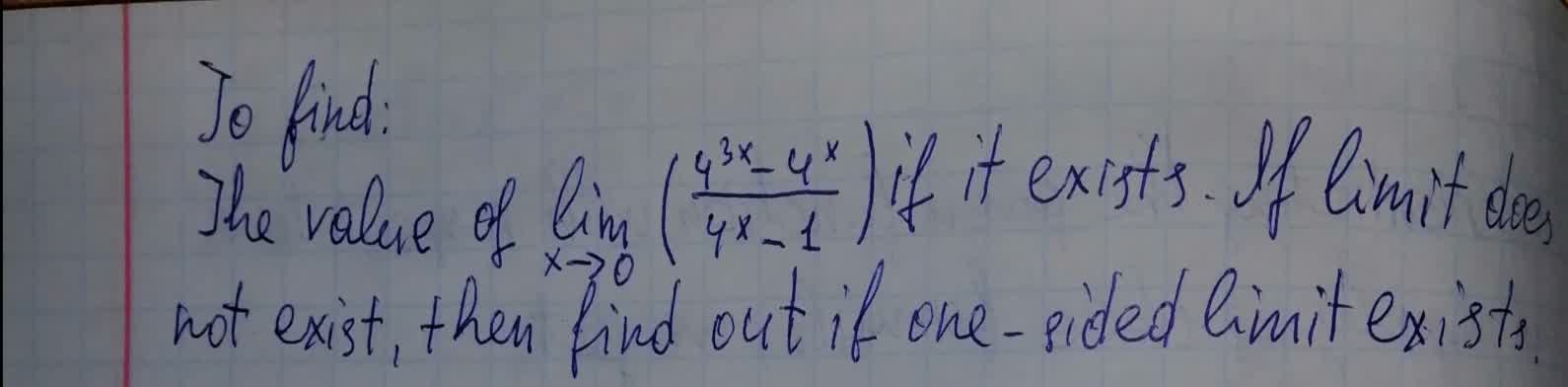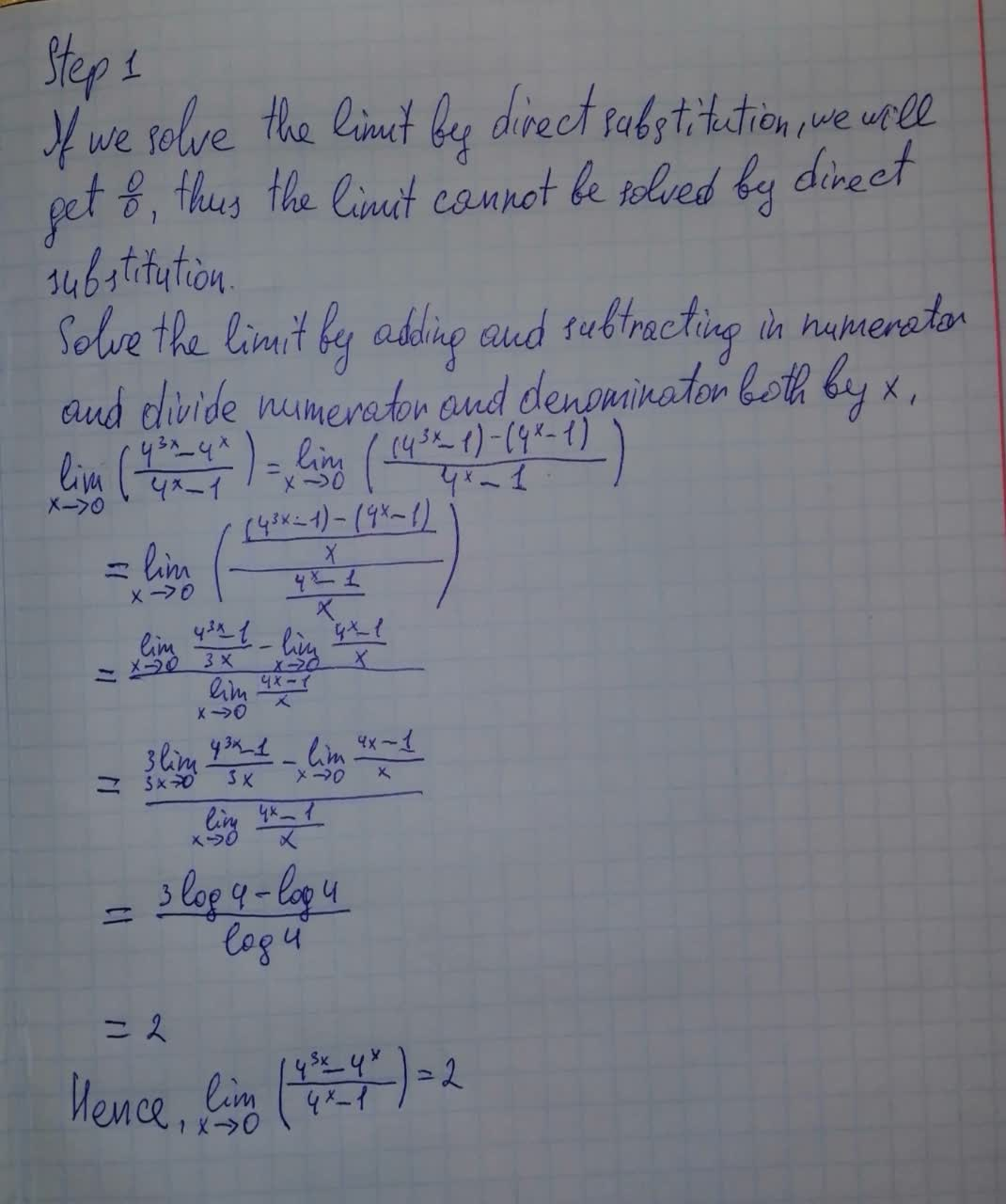Question# To find:The value of lim_{x \rightarrow 0} (\frac{4^{3x}-4^x}{4^x-1}) if it exists. If limit does not exist ,then find out if one-sided limit exists

Conic sections
ANSWEREDTo find:
The value of $$\displaystyle\lim_{{{x}\rightarrow{0}}}{\left({\frac{{{4}^{{{3}{x}}}-{4}^{{x}}}}{{{4}^{{x}}-{1}}}}\right)}$$ if it exists. If limit does not exist , then find out if one-sided limit exists.